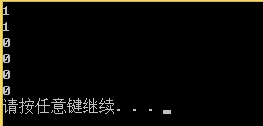# 布隆过滤器BloomFilter

1. 原理
Bloom Filter 实际上是由一个很长的二进制向量和一系列随机映射函数组成，布隆过滤器可以用于检索一个元素是否在一个集合中。它的优点是空间效率和查询时间都远远超过一般的算法，缺点是有一定的误识别率（假正例False positives，即Bloom Filter报告某一元素存在于某集合中，但是实际上该元素并不在集合中）和删除困难，但是没有识别错误的情形（即假反例False negatives，如果某个元素确实没有在该集合中，那么Bloom Filter 是不会报告该元素存在于集合中的，所以不会漏报）。
Bloom Filter 是一种空间效率很高的随机数据结构，Bloom filter 可以看做是对 bit-map 的扩展, 它的原理是：

(误判补救方法是：再建立一个小的白名单，存储那些可能被误判的信息。)

2.代码实现

//BloomFilter.h
#pragma once
#include"BitSet.h"

struct _HashFunc1
{
size_t BKDRHash(const char *str)
{
register size_t hash = 0;
while (size_t ch = (size_t)*str++)
{
hash = hash * 131 + ch;   // 也可以乘以31、131、1313、13131、131313..
}
return hash;
}
size_t operator() (const std::string& key)
{
return BKDRHash(key.c_str());
}
};

struct _HashFunc2
{
size_t SDBMHash(const char* str)
{
register size_t hash = 0;
while (size_t ch = (size_t)*str++)
{
hash = 65599 * hash + ch;
//hash = (size_t)ch + (hash << 6) + (hash << 16) - hash;
}
return hash;
}

size_t operator()(const std::string& key)
{
return SDBMHash(key.c_str());
}
};

struct _HashFunc3
{
size_t RSHash(const char* str)
{
register size_t hash = 0;
size_t magic = 63689;
while (size_t ch = (size_t)*str++)
{
hash = hash * magic + ch;
magic *= 378551;
}
return hash;
}

size_t operator()(const std::string& key)
{
return RSHash(key.c_str());
}
};

template<class K=string, class HashFunc1 = _HashFunc1, class HashFunc2 = _HashFunc2, class HashFunc3 = _HashFunc3>
class BloomFilter
{
public:
BloomFilter(size_t num)
:_bs(num * 5)
,_range(num*5)
{}
void Set(const K& key)
{
size_t hash1 = HashFunc1()(key);
size_t hash2 = HashFunc1()(key);
size_t hash3 = HashFunc1()(key);

_bs.Set(hash1%_range);
_bs.Set(hash2%_range);
_bs.Set(hash3%_range);
}

//void Reset(const K& key)BloomFilter不可以进行取消设置，因为会有附带影响
bool Test(const K& key)
{
size_t hash1 = HashFunc1()(key);
if (_bs.Test(hash1%_range)==false)
{
return false;
}
size_t hash2 = HashFunc1()(key);
if (_bs.Test(hash2%_range) == false)
{
return false;
}
size_t hash3 = HashFunc1()(key);
if (_bs.Test(hash3%_range) == false)
{
return false;
}
return true;//不准确
}

protected:
BitSet _bs;
size_t _range;
};
//test.cpp
#include<iostream>
#include<utility>
#include<string>
#include"bloomfilter.h"
using namespace std;
void TestBloomFilter()
{
BloomFilter<> bf(10);
bf.Set("sort");
bf.Set("bloom");
bf.Set("filter");
bf.Set("bloomfilter");
bf.Set("range");

cout << bf.Test("sort") << endl;
cout << bf.Test("bloom") << endl;

cout << bf.Test("sort1") << endl;
cout << bf.Test("debug") << endl;
cout << bf.Test("insert") << endl;
cout << bf.Test("end") << endl;
}
int main()
{
TestBloomFilter();
system("pause");
return 0;
}©️2019 CSDN 皮肤主题: 编程工作室 设计师: CSDN官方博客